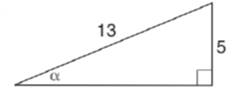Chapter 11.3, Problem 5EElementary Geometry For College St...

7th Edition
Alexander + 2 others
ISBN: 9781337614085

Solutions

Chapter
SectionElementary Geometry For College St...

7th Edition
Alexander + 2 others
ISBN: 9781337614085
Textbook Problem

In Exercises 5 to 10, find the value (or expression) for each of the six trigonometric ratios of angle α . Use the Pythagorean Theorem as needed.To determine

To find:

The value for each of the six trigonometric ratios of angle α.

Explanation

Given:

The given figure is

Definition used:

The six trigonometric ratios:

Calculation:

Let the value of the unknown side in the figure be x.

In a right triangle, (hypotenuse)2=(opposite)2+(adjacent)2

(13)2=52+x2169=25+x216925=x2144=x2144=x12=x

Thus we have

Still sussing out bartleby?

Check out a sample textbook solution.

See a sample solution

The Solution to Your Study Problems

Bartleby provides explanations to thousands of textbook problems written by our experts, many with advanced degrees!

Get Started

In Exercises 7-22, evaluate the expression. 22. 62.561.961.4

Applied Calculus for the Managerial, Life, and Social Sciences: A Brief Approach

In Exercises 1728, use the logarithm identities to obtain the missing quantity.

Finite Mathematics and Applied Calculus (MindTap Course List)

Evaluate ∫16t3 ln t dt. 4t4 + C 4t3 ln t − 16t3 + C 4t4 − t3 ln t + C 4t4 ln t − t4 + C

Study Guide for Stewart's Single Variable Calculus: Early Transcendentals, 8th